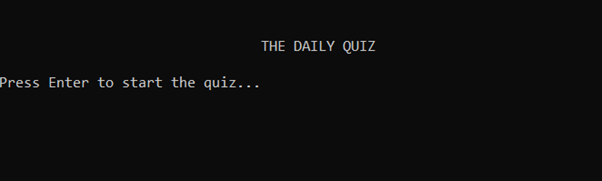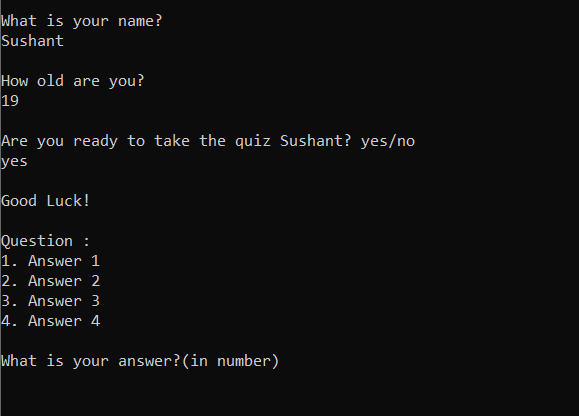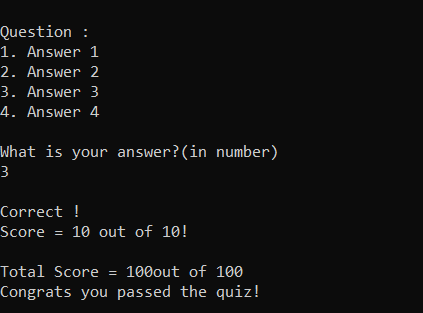Related Articles
Quiz Game in C++
• Difficulty Level : Medium
• Last Updated : 25 Feb, 2021

In this article, the task is to create a quiz game where the user will be asked questions and the result of each question whether it is correct or wrong with the updated score will be shown.

Below is the implementation of the same:

## C++

 `// C++ program for the above approach``#include ``#include ``using` `namespace` `std;`` ` `int` `Guess;``int` `Total;`` ` `// Question Class``class` `Question {``private``:``    ``string Question_Text;``    ``string Answer_1;``    ``string Answer_2;``    ``string Answer_3;``    ``string Answer_4;``    ``int` `Correct_Answer;``    ``int` `Question_Score;`` ` `public``:``    ``// Setter Function``    ``void` `setValues(string, string,``                   ``string, string,``                   ``string, ``int``, ``int``);`` ` `    ``// Function to ask questions``    ``void` `askQuestion();``};`` ` `// Driver code``int` `main()``{``    ``cout << ``"\n\n\t\t\t\tTHE DAILY QUIZ"``         ``<< endl;``    ``cout << ``"\nPress Enter to start "``         ``<< ``"the quiz... "` `<< endl;`` ` `    ``// Input``    ``cin.get();`` ` `    ``string Name;``    ``int` `Age;`` ` `    ``// Input the details``    ``cout << ``"What is your name?"``         ``<< endl;``    ``cin >> Name;``    ``cout << endl;`` ` `    ``cout << ``"How old are you?"``         ``<< endl;``    ``cin >> Age;``    ``cout << endl;`` ` `    ``string Respond;``    ``cout << ``"Are you ready to take"``         ``<< ``" the quiz "` `<< Name``         ``<< ``"? yes/no"` `<< endl;``    ``cin >> Respond;`` ` `    ``if` `(Respond == ``"yes"``) {``        ``cout << endl;``        ``cout << ``"Good Luck!"` `<< endl;``    ``}``    ``else` `{``        ``cout << ``"Okay Good Bye!"` `<< endl;``        ``return` `0;``    ``}`` ` `    ``// Objects of Question Class``    ``Question q1;``    ``Question q2;``    ``Question q3;``    ``Question q4;``    ``Question q5;``    ``Question q6;``    ``Question q7;``    ``Question q8;``    ``Question q9;``    ``Question q10;`` ` `    ``// 3 is the position of``    ``// correct answer``    ``q1.setValues(``"Question : "``, ``"Answer 1"``,``                 ``"Answer 2"``, ``"Answer 3"``,``                 ``"Answer 4"``, 3, 10);``    ``q2.setValues(``"Question : "``, ``"Answer 1"``,``                 ``"Answer 2"``, ``"Answer 3"``,``                 ``"Answer 4"``, 3, 10);``    ``q3.setValues(``"Question : "``, ``"Answer 1"``,``                 ``"Answer 2"``, ``"Answer 3"``,``                 ``"Answer 4"``, 3, 10);``    ``q4.setValues(``"Question : "``, ``"Answer 1"``,``                 ``"Answer 2"``, ``"Answer 3"``,``                 ``"Answer 4"``, 3, 10);``    ``q5.setValues(``"Question : "``, ``"Answer 1"``,``                 ``"Answer 2"``, ``"Answer 3"``,``                 ``"Answer 4"``, 3, 10);``    ``q6.setValues(``"Question : "``, ``"Answer 1"``,``                 ``"Answer 2"``, ``"Answer 3"``,``                 ``"Answer 4"``, 3, 10);``    ``q7.setValues(``"Question : "``, ``"Answer 1"``,``                 ``"Answer 2"``, ``"Answer 3"``,``                 ``"Answer 4"``, 3, 10);``    ``q8.setValues(``"Question : "``, ``"Answer 1"``,``                 ``"Answer 2"``, ``"Answer 3"``,``                 ``"Answer 4"``, 3, 10);``    ``q9.setValues(``"Question : "``, ``"Answer 1"``,``                 ``"Answer 2"``, ``"Answer 3"``,``                 ``"Answer 4"``, 3, 10);``    ``q10.setValues(``"Question : "``, ``"Answer 1"``,``                  ``"Answer 2"``, ``"Answer 3"``,``                  ``"Answer 4"``, 3, 10);`` ` `    ``q1.askQuestion();``    ``q2.askQuestion();``    ``q3.askQuestion();``    ``q4.askQuestion();``    ``q5.askQuestion();``    ``q6.askQuestion();``    ``q7.askQuestion();``    ``q8.askQuestion();``    ``q9.askQuestion();``    ``q10.askQuestion();`` ` `    ``// Display the total score``    ``cout << ``"Total Score = "` `<< Total``         ``<< ``"out of 100"` `<< endl;`` ` `    ``// Display the results`` ` `    ``// If the player pass the quiz``    ``if` `(Total >= 70) {``        ``cout << ``"Congrats you passed the"``             ``<< ``" quiz!"` `<< endl;``    ``}`` ` `    ``// Otherwise``    ``else` `{``        ``cout << ``"Alas! You failed the quiz."``             ``<< endl;``        ``cout << ``"Better luck next time."``             ``<< endl;``    ``}``    ``return` `0;``}`` ` `// Function to set the values of``// the questions``void` `Question::setValues(``    ``string q, string a1,``    ``string a2, string a3,``    ``string a4, ``int` `ca, ``int` `pa)``{``    ``Question_Text = q;``    ``Answer_1 = a1;``    ``Answer_2 = a2;``    ``Answer_3 = a3;``    ``Answer_4 = a4;``    ``Correct_Answer = ca;``    ``Question_Score = pa;``}`` ` `// Function to ask questions``void` `Question::askQuestion()``{``    ``cout << endl;`` ` `    ``// Print the questions``    ``cout << Question_Text << endl;``    ``cout << ``"1. "` `<< Answer_1 << endl;``    ``cout << ``"2. "` `<< Answer_2 << endl;``    ``cout << ``"3. "` `<< Answer_3 << endl;``    ``cout << ``"4. "` `<< Answer_4 << endl;``    ``cout << endl;`` ` `    ``// Display the answer``    ``cout << ``"What is your answer?(in number)"``         ``<< endl;``    ``cin >> Guess;`` ` `    ``// If the answer is correct``    ``if` `(Guess == Correct_Answer) {``        ``cout << endl;``        ``cout << ``"Correct !"` `<< endl;`` ` `        ``// Update the correct score``        ``Total = Total + Question_Score;``        ``cout << ``"Score = "` `<< Question_Score``             ``<< ``" out of "``             ``<< Question_Score``             ``<< ``"!"` `<< endl;``        ``cout << endl;``    ``}`` ` `    ``// Otherise``    ``else` `{``        ``cout << endl;``        ``cout << ``"Wrong !"` `<< endl;``        ``cout << ``"Score = 0"``             ``<< ``" out of "``             ``<< Question_Score``             ``<< ``"!"` `<< endl;``        ``cout << ``"Correct answer = "``             ``<< Correct_Answer``             ``<< ``"."` `<< endl;``        ``cout << endl;``    ``}``}`

Output:Want to learn from the best curated videos and practice problems, check out the C++ Foundation Course for Basic to Advanced C++ and C++ STL Course for foundation plus STL.  To complete your preparation from learning a language to DS Algo and many more,  please refer Complete Interview Preparation Course.

My Personal Notes arrow_drop_up